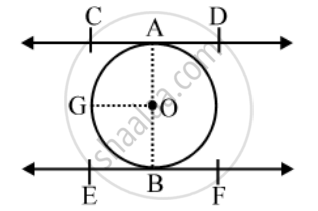# Prove that the Line Segment Joining the Point of Contact of Two Parallel Tangents to a Circle is a Diameter of the Circle. - Mathematics

Sum

Prove that the line segment joining the point of contact of two parallel tangents to a circle is a diameter of the circle.

#### Solution

Given: CD and EF are two parallel tangents at the points A and B of a circle with centre O.To prove: AOB is a diameter of the circle.

Construction: Join OA and OB.

Draw OG || CD

Proof: OG || CD and AO cuts them.

∴ ∠CAO + ∠GOA = 180°

⇒ ∠GOA = 180°

⇒ ∠GOA = 90°

Similarly, ∠GOB = 90°

∴ ∠GOA + ∠GOB = (90° + 90°) = 180°

⇒ AOB is a straight line

Hence, AOB is a diameter of the circle with centre O.

Concept: Concept of Circle - Centre, Radius, Diameter, Arc, Sector, Chord, Segment, Semicircle, Circumference, Interior and Exterior, Concentric Circles
Is there an error in this question or solution?